# 2D Line Plot in MATLAB

• Last Updated : 29 May, 2021

‘2D’ stands for 2-dimensional and a 2D line is a line that is moved in 2-dimensions. A line in 2D means that we could move in forward and backward direction but also in any direction like left, right, up, down.

In MATLAB we have a function named plot() which allows us to plot a line in 2 directions.

Syntax:

plot(X,Y)

where X and Y represent the x and the y axis of the plane. The X and Y both are can be vectors or matrices but there are some conditions to plot the graph and these conditions are mentioned below:

Condition 1: If X and Y both are vectors then they must be of equal length.

Condition 2: If X and Y both are Matrices then they must be of equal size.

Condition 3: If one of X or Y is a vector and the other is a matrix, then the matrix must have dimensions such that one of its dimensions equals the vector length.

Condition 4: If one is a scalar and the other is either a scalar or a vector, then discrete points must be plotted.

Now let’s move to some examples.

Example 1: Draw a simple line:

## MATLAB

 `% coordinates of the x-axis` `x=[10,20,30,40,50]`   `% coordinates of the y-axis` `y=[100,200,300,400,500]`   `% plot function is used to plot the ` `% line according to the coordinates` `plot(x,y)` `  `  `% to put grid on the graph` `grid on`

Output :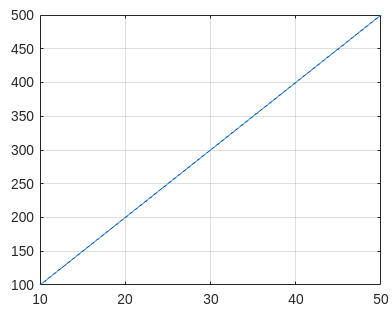Example 2: Draw a line with only 1 axis coordinates as input:

Note: If you give only 1 axis then the plot() function takes it as coordinates of the y-axis and by default gives values to the x-axis starting from 1, 2, 3, up to y coordinates.

## MATLAB

 `% coordinates of axis` `y=[100,200,300,400,500]`   `% plot function is used to plot the ` `% line according to the coordinates` `plot(x,y)`   `% to put grid on the graph` `grid on`

Output :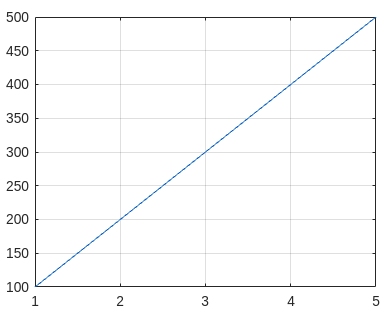Example 3: Draw more than 1 line on the same graph with axis names:

## MATLAB

 `% coordinates of x-axis` `x=[10,20,30,40,50]`   `% coordinates of y-axis of line 1` `% represented by blue color` `y1=[100,500,200,100,0]`   `% coordinates of y-axis of line 2` `% represented by red color` `y2=[400,100,0,200,300]`   `% coordinates of y-axis of line 3` `% represented by yellow color` `y3=[200,300,400,100,500]`   `% plot function to plot the lines on graph` `plot(x,y1,x,y2,x,y3)`   `% to add grid on graph` `grid on`   `% name of x axis` `xlabel(``'x'``)` `  `  `% name of y axis` `ylabel(``'y'``)`

Output :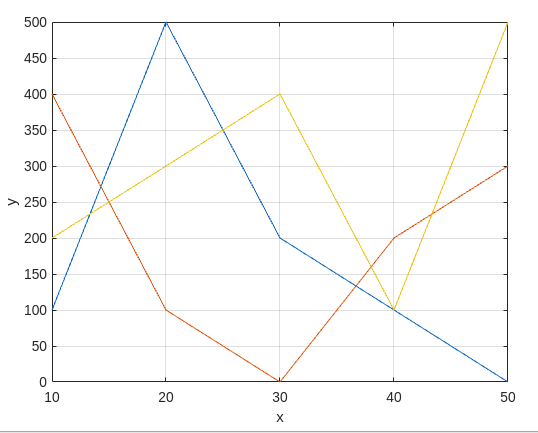Example 4: Now the value of the y-axis is given as matrix instead of vector:

## MATLAB

 `% coordinates of x-axis` `x=[1,2,3,4,5]`   `% coordinates of y-axis in form of matrix` `% magic(n) matrix is a n*n matrix` `% in which value scattered from 1 to n^2` `% with equal row and columns sum` `y=magic(5)`   `% plot function` `plot(x,y)`   `% to add grid` `grid on`   `% add name on axis` `xlabel(``'x'``)` `ylabel(``'y'``)`

Output: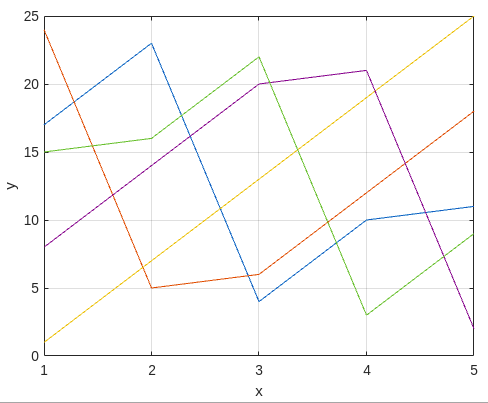Example 5: Now we plot the graph by using 2 y-axis one is on the left side another is on the right side.

## MATLAB

 `% coordinates of x-axis` `x=[1,2,3,4,5]`   `% coordinates of y-axis ` `y=[50,40,30,20,10]`   `% assigning left side to the above` `% coordinates` `yyaxis left`   `% plot graph of left y-axis ` `plot(x,y)` `  `  `% coordinates of y-axis` `y=[10,20,30,40,50]`   `% assigning right side to the above ` `% coordinates` `yyaxis right`   `% plot graph of right y-axis ` `plot(x,y)`   `% put grid on graph` `grid on`   `% name of x-axis` `xlabel(``'x'``)` `  `  `% name of left side y coordinates` `yyaxis left` `ylabel(``'Left Side'``)` `  `  `% name of right side y coordinates` `yyaxis right` `ylabel(``'Right Side'``)`

Output: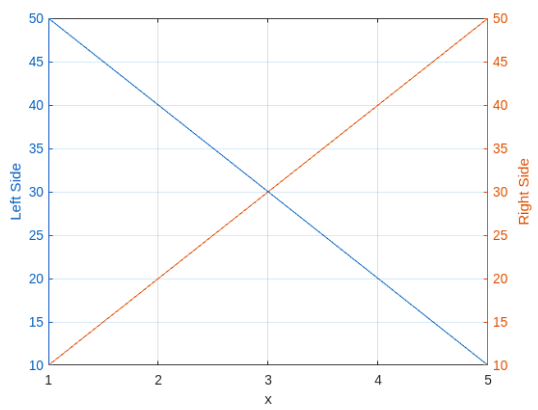My Personal Notes arrow_drop_up
Recommended Articles
Page :